Main Content

# jc_0802: Prohibited use of implicit type casting in Stateflow

• NA-MAAB — a

• JMAAB — a

All

## Rule

### Sub ID a

All operations, including substitution, comparison, arithmetic, etc., shall be performed between variables of the same data type.

The data type of the actual arguments and the formal arguments in a function call shall be the same.

Custom Parameter

Not Applicable

Example — Correct

Variables use the same data type for calculations.

Example: Comparison operation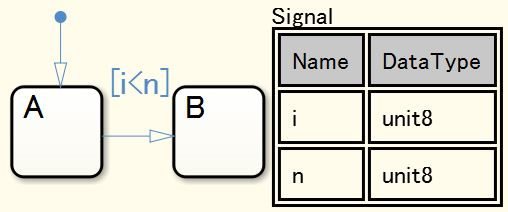Example: Arithmetic operations and assignment operations (compound expressions)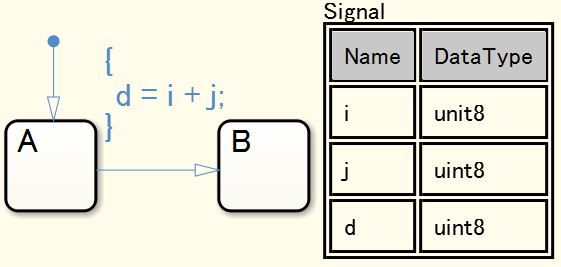Variables have different data types but are explicitly typecast before calculation.

Example: Comparison operation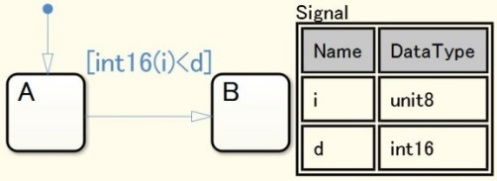Example: Arithmetic operations and assignment operations (compound expressions)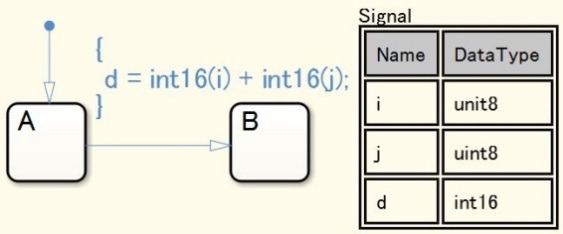The data type of actual arguments and formal arguments in the function call are the same.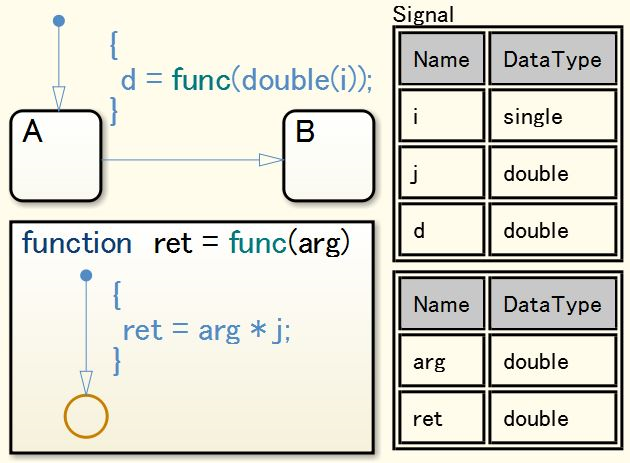Example — Incorrect

Variables use different data types for calculations.

Example: Comparison operation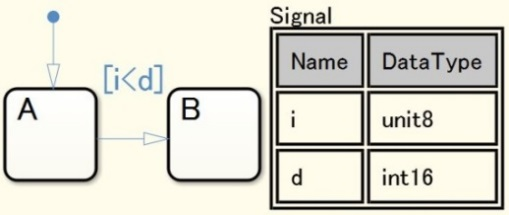Example: Arithmetic operations and assignment operations (compound expressions)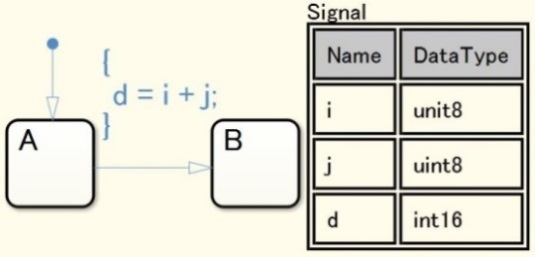Calculations are performed between unsigned integer type variables and signed integers.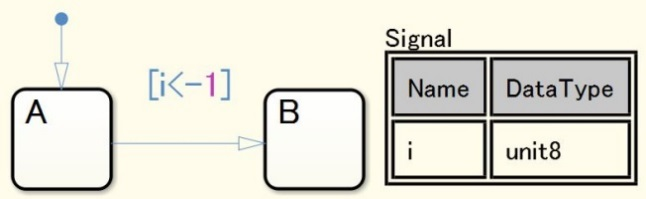The data type of actual arguments and formal arguments in the function call are different.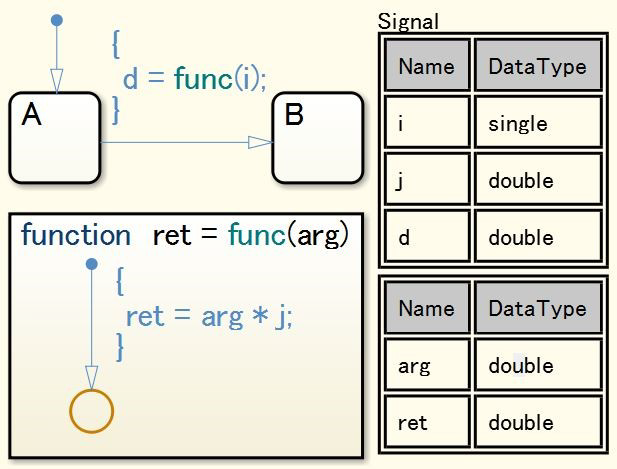## Rationale

Sub ID a:

• Implicit data type conversion can produce unexpected results.

## Verification

Model Advisor check: Check for implicit type casting in Stateflow (Simulink Check)

R2020a

## See Also

Introduced in R2020a

## Support

#### Model-Based Design for Embedded Control Systems

Download white paper